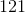# ISEE Middle Level Math : Data Analysis

## Example Questions

### Example Question #5 : Mode

Find the mode in this set of numbers:

2521, 2512, 2525, 2552, 2551, 2525, 2515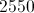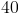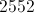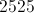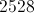Explanation:

First, order the numbers from least to greatest: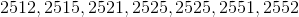Identify the most recurring number: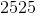### Example Question #441 : Isee Middle Level (Grades 7 8) Mathematics Achievement

Find the mode in this set of numbers:

3343, 4434, 4334, 3343, 4343, 3434, 3334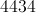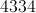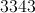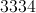Explanation:

Identify the most recurring number: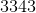### Example Question #11 : Mode

Find the mode in this set of numbers:

6363, 6336, 6633, 6333, 6363, 6663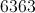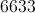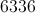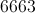Explanation:

Find the most recurring number: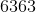### Example Question #12 : How To Find Mode

Find the mode in this set of numbers:

5855, 5588, 5585, 5858, 5885, 5558, 5885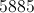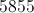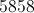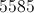Explanation:

Find the most recurring number: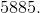.

### Example Question #11 : How To Find Mode

Find the mode in this set of numbers: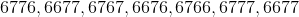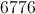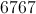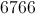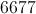Explanation:

Find the most recurring number: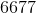### Example Question #14 : Mode

Give the mode(s) of this data set: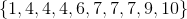Explanation:

The mode of a data set is the value or values that appears the most frequently. 4 and 7 appear three times each, and no other value appears more than once. 4 and 7 are therefore the two modes.

### Example Question #13 : How To Find Mode

Give the mode(s) of this data set: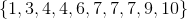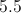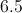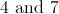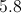Explanation:

The mode of a data set is the value or values that appears the most frequently. 7 appears three times, 4 appears two times, and no other value appears more than once. 7 is therefore the only mode.

### Example Question #131 : Data Analysis

Find the mode in this set of numbers:

9080, 9008, 9800, 9099, 9009, 9090, 9008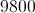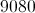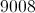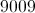Explanation:

Find the most recurring number: 9008.

### Example Question #14 : How To Find Mode

Subtract the range from the mode in this set of numbers:

9080, 9008, 9800, 9099, 9009, 9090, 9008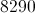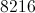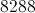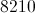Explanation:

First, order the numbers from least to greatest: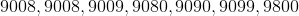Then, find the range (subtract the smallest number from the greatest):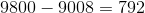Then, find the mode (identify the most recurring number):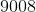Finally, subtract the range from the mode: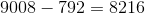### Example Question #442 : Isee Middle Level (Grades 7 8) Mathematics Achievement

Find the mode in this set of numbers:

212, 121, 112, 221, 122, 121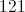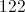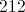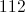Identify the most recurring number: